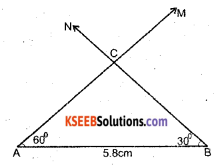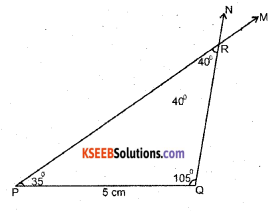# KSEEB Solutions for Class 7 Maths Chapter 10 Practical Geometry Ex 10.4

Students can Download Chapter 10 Practical Geometry Ex 10.4, Question and Answers, Notes Pdf, KSEEB Solutions for Class 7 Maths, Karnataka State Board Solutions help you to revise complete Syllabus and score more marks in your examinations.

## Karnataka State Syllabus Class 7 Maths Chapter 10 Practical Geometry Ex 10.4

Question 1.
Construct ∆ ABC, given m∠A= 30° and m∠B = 30° and AB = 5.8 cm.
Solution:Steps of Construction.

1. Draw a line segment AB of length 5.8 cm, at A draw a ray AM making an angle of 60° with AB.
2. At ‘B’ draw a ray ABN making an angle of 30° with BA.
3. Mark the point of intersection of two rays as ‘C’. Now we get the A ABC which is required.Question 2.
Construct ∆ PQR if PQ = 5 cm, m∠PQR = 105° and m∠QRP = 40°. (Hint Recall angle-sum property of a triangle).
Solution:By angle-sum property of a triangle ∠RPQ + ∠PQR + ∠QRP = 180°
∠RPQ + 105° + 40° = 180°
∠RPQ + 145° = 180°
∴ ∠RPQ = 180° – 145° = 35°,

a

Steps of Construction

1. Draw a line segment PQ of length 5 cm. At P draw a ray PM making an angle of 35° with PQ.
2. At Q draw a ray QN making an angle of 105° with QP.
3. Mark the point of intersection of two rays as R. We get the required P Q R triangle.Question 3.
Examine whether you can construct ∆ DEF such that E F = 7.2 cm. m∠E = 110° and m∠F = 80°. Justify your answer.
Solution:
∠E + ∠F = 110°+ 80°= 190°
This triangle is not possible to construct because the measures of two angles exceed 180°.
The sum of three angles of a triangle is always equal to 180°.
So it is not possible.

a